Home
Hostname: page-component-79b67bcb76-ncjtf Total loading time: 0.19 Render date: 2021-05-12T12:35:50.077Z Has data issue: true Feature Flags: { "shouldUseShareProductTool": true, "shouldUseHypothesis": true, "isUnsiloEnabled": true, "metricsAbstractViews": false, "figures": false, "newCiteModal": false, "newCitedByModal": true, "newEcommerce": true }Compositio Mathematica

# Diophantine properties of nilpotent Lie groups

Published online by Cambridge University Press:  13 January 2015

Corresponding

## Abstract

A finitely generated subgroup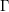${\rm\Gamma}$ of a real Lie group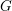$G$ is said to be Diophantine if there is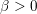${\it\beta}>0$ such that non-trivial elements in the word ball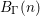$B_{{\rm\Gamma}}(n)$ centered at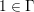$1\in {\rm\Gamma}$ never approach the identity of$G$ closer than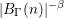$|B_{{\rm\Gamma}}(n)|^{-{\it\beta}}$. A Lie group$G$ is said to be Diophantine if for every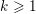$k\geqslant 1$ a random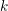$k$-tuple in$G$ generates a Diophantine subgroup. Semi-simple Lie groups are conjectured to be Diophantine but very little is proven in this direction. We give a characterization of Diophantine nilpotent Lie groups in terms of the ideal of laws of their Lie algebra. In particular we show that nilpotent Lie groups of class at most$5$, or derived length at most$2$, as well as rational nilpotent Lie groups are Diophantine. We also find that there are non-Diophantine nilpotent and solvable (non-nilpotent) Lie groups.

## MSC classification

Type
Research Article
Information
Compositio Mathematica , June 2015 , pp. 1157 - 1188

## References

Bass, H., The degree of polynomial growth of finitely generated nilpotent groups, Proc. Lond. Math. Soc. (3) 25 (1972), 603614.CrossRefGoogle Scholar
Bombieri, E. and Gubler, W., Heights in Diophantine geometry, New Mathematical Monographs, vol. 4 (Cambridge University Press, Cambridge, 2006).Google Scholar
Bourgain, J. and Gamburd, A., On the spectral gap for finitely generated subgroups of SU(2), Invent. Math. 171 (2008), 83121.CrossRefGoogle Scholar
Breuillard, E., Equidistribution of dense subgroups on nilpotent Lie groups, Ergodic Theory Dynam. Systems 30 (2010), 131150.CrossRefGoogle Scholar
Breuillard, E., Heights on SL2 and free subgroups, in Geometry, rigidity, and group actions, Chicago Lectures in Mathematics (University Chicago Press, Chicago, IL, 2011), 455493.Google Scholar
Breuillard, E., Geometry of locally compact groups of polynomial growth and shape of large balls, Groups Geom. Dyn. 8 (2014), 669732.CrossRefGoogle Scholar
Corwin, L. J. and Greenleaf, F. P., Representations of nilpotent Lie groups and their applications. Part I, inBasic theory and examples, Cambridge Studies in Advanced Mathematics, vol. 18 (Cambridge University Press, Cambridge, 1990).Google Scholar
Dodson, M. M., Hausdorff dimension, lower order and Khintchine’s theorem in metric Diophantine approximation, J. Reine Angew. Math. 432 (1992), 6976.Google Scholar
Fulton, W. and Harris, J., Representation theory, A first course, readings in mathematics, Graduate Texts in Mathematics, vol. 129 (Springer, New York, NY, 1991).Google Scholar
Gamburd, A., Hoory, S., Shahshahani, M., Shalev, A. and Virág, B., On the girth of random Cayley graphs, Random Structures Algorithms 35 (2009), 100117.CrossRefGoogle Scholar
Gamburd, A., Jakobson, D. and Sarnak, P., Spectra of elements in the group ring of SU(2), J. Eur. Math. Soc. (JEMS) 1 (1999), 5185.CrossRefGoogle Scholar
Ganzburg, M. I., Polynomial inequalities on measurable sets and their applications. II. Weighted measures, J. Approx. Theory 106 (2000), 77109.CrossRefGoogle Scholar
Ganzburg, M. and Brudnyi, J., On an extremal problem for polynomials in n variables, Math. USSR Izv. 7 (1973), 345356.Google Scholar
Gromov, M., Groups of polynomial growth and expanding maps, Publ. Math. Inst. Hautes Études Sci. 53 (1981), 5373.CrossRefGoogle Scholar
Guivarc’h, Y., Croissance polynomiale et périodes des fonctions harmoniques, Bull. Soc. Math. France 101 (1973), 353379.Google Scholar
Hall, M. Jr, The theory of groups (Chelsea Publishing, New York, NY, 1976).Google Scholar
Jarník, V., Über die simultanen diophantischen approximationen, Math. Z. 33 (1931), 505543.CrossRefGoogle Scholar
Johnson, M., Standard tableaux and Klyachko’s theorem on Lie representations, J. Combin. Theory Ser. A 114 (2007), 151158.CrossRefGoogle Scholar
Kaloshin, V. and Rodnianski, I., Diophantine properties of elements of SO(3), Geom. Funct. Anal. 11 (2001), 953970.CrossRefGoogle Scholar
Kleinbock, D. Y. and Margulis, G. A., Flows on homogeneous spaces and Diophantine approximation on manifolds, Ann. of Math. (2) 148 (1998), 339360.CrossRefGoogle Scholar
Kleinbock, D. and Tomanov, G., Flows on S-arithmetic homogeneous spaces and applications to metric Diophantine approximation, Comment. Math. Helv. 82 (2007), 519581.CrossRefGoogle Scholar
Kljačko, A. A., Lie elements in a tensor algebra, Sibirsk. Mat. Zh. 15 (1974), 12961304, 1430.Google Scholar
Kovács, L. G. and Stöhr, R., A combinatorial proof of Klyachko’s theorem on Lie representations, J. Algebraic Combin. 23 (2006), 225230.CrossRefGoogle Scholar
Kraskiewicz, W. and Weyman, J., Algebra of coinvariants and the action of coxeter elements, Bayreuth. Math. Schr. 63 (2001), 265284.Google Scholar
Magnus, W., Karrass, A. and Solitar, D., Combinatorial group theory: Presentations of groups in terms of generators and relations, revised edition (Dover, New York, NY, 1976).Google Scholar
Pansu, P., Croissance des boules et des géodésiques fermées dans les nilvariétés, Ergodic Theory Dynam. Systems 3 (1983), 415445.CrossRefGoogle Scholar
Raghunathan, M. S., Discrete subgroups of Lie groups (Springer, 1972).CrossRefGoogle Scholar
Remez, E., Sur une propriétée extrémale des polynômes de Tchebychef, Comm. Inst. Sci. Kharkov 13 (1936), 9395.Google Scholar
Reutenauer, C., Free Lie algebras (Oxford University Press, 1993).Google Scholar
Sarnak, P., Some applications of modular forms, Cambridge Tracts in Mathematics, vol. 99 (Cambridge University Press, 1990).CrossRefGoogle Scholar
Serre, J.-P., Lie algebras and Lie groups, Lecture Notes in Mathematics, vol. 1500, second edition (corrected fifth printing) (Springer, Berlin, 2006).Google Scholar
Thom, A., Convergent sequences in discrete groups, Canad. Math. Bull. 56 (2013), 424433.CrossRefGoogle Scholar
Thrall, R. M., On symmetrized Kronecker powers and the structure of the free Lie ring, Amer. J. Math. 64 (1942), 371388.CrossRefGoogle Scholar
Varjú, P. P., Diophantine property in the group of affine transformation of the line, Preprint (2012), arXiv:1211.3647 [math.GR].Google Scholar
Witt, E., Treue Darstellung Liescher Ringe, J. Reine Angew. Math. 177 (1937), 152160.Google ScholarYou have Access

# Send article to Kindle

Note you can select to send to either the @free.kindle.com or @kindle.com variations. ‘@free.kindle.com’ emails are free but can only be sent to your device when it is connected to wi-fi. ‘@kindle.com’ emails can be delivered even when you are not connected to wi-fi, but note that service fees apply.

Find out more about the Kindle Personal Document Service.

Diophantine properties of nilpotent Lie groups
Available formats
×

# Send article to Dropbox

To send this article to your Dropbox account, please select one or more formats and confirm that you agree to abide by our usage policies. If this is the first time you use this feature, you will be asked to authorise Cambridge Core to connect with your <service> account. Find out more about sending content to Dropbox.

Diophantine properties of nilpotent Lie groups
Available formats
×

# Send article to Google Drive

To send this article to your Google Drive account, please select one or more formats and confirm that you agree to abide by our usage policies. If this is the first time you use this feature, you will be asked to authorise Cambridge Core to connect with your <service> account. Find out more about sending content to Google Drive.

Diophantine properties of nilpotent Lie groups
Available formats
×
×